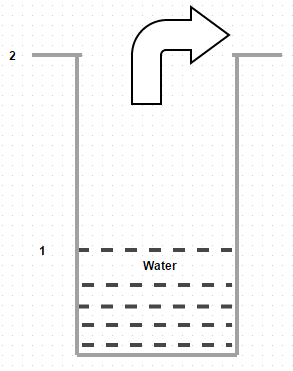Courses

# Test: Concentrations, Velocities And Fluxes

## 10 Questions MCQ Test Mass Transfer | Test: Concentrations, Velocities And Fluxes

Description
This mock test of Test: Concentrations, Velocities And Fluxes for Chemical Engineering helps you for every Chemical Engineering entrance exam. This contains 10 Multiple Choice Questions for Chemical Engineering Test: Concentrations, Velocities And Fluxes (mcq) to study with solutions a complete question bank. The solved questions answers in this Test: Concentrations, Velocities And Fluxes quiz give you a good mix of easy questions and tough questions. Chemical Engineering students definitely take this Test: Concentrations, Velocities And Fluxes exercise for a better result in the exam. You can find other Test: Concentrations, Velocities And Fluxes extra questions, long questions & short questions for Chemical Engineering on EduRev as well by searching above.
QUESTION: 1

### The ratio of mass concentration of species A to the total mass density of the mixture is known as

Solution:

Explanation: It is defined as p a/p.

QUESTION: 2

### For a binary mixture of species A and B, the mass average velocity is defined as

Solution:

Explanation: p a V a + p b V b/ p a + p = p a V a + p b V b/ p.

QUESTION: 3

### The ratio of number of moles of species A to the total number of moles of the mixture is known as

Solution:

Explanation: It is defined as n a/n.

QUESTION: 4

A binary mixture of oxygen and nitrogen with partial pressures in the ratio 0.21 and 0.79 is contained in a vessel at 300 K. If the total pressure of the mixture is 1 * 10 5 N/m2, find molar concentration of oxygen

Solution:

Explanation: 0.21 * 10 5/8341 * 300 = 8.42 * 10 -3 kg mol/m3.

QUESTION: 5

For a binary mixture of species A and B, the molar average velocity is defined as

Solution:

Explanation: n A V A + n B V B/n A + n B = n A V A + n B V B/n.

QUESTION: 6

Given diagram shows diffusion of water vapor through air. Identify the correct statementSolution:

Explanation: The slight movement of air over the top of the tube does not bring about any change in the concentration profile of air.

QUESTION: 7

A binary mixture of oxygen and nitrogen with partial pressures in the ratio 0.21 and 0.79 is contained in a vessel at 300 K. If the total pressure of the mixture is 1 * 10 5 N/m2, find overall mass density

Solution:

Explanation: For oxygen = 0.269 kg/m3 and for nitrogen = 0.887 kg/m3.

QUESTION: 8

A binary mixture of oxygen and nitrogen with partial pressures in the ratio 0.21 and 0.79 is contained in a vessel at 300 K. If the total pressure of the mixture is 1 * 10 5 N/m2, find molar fraction of nitrogen

Solution:

Explanation: Molar fraction is equal to the partial pressure.

QUESTION: 9

A binary mixture of oxygen and nitrogen with partial pressures in the ratio 0.21 and 0.79 is contained in a vessel at 300 K. If the total pressure of the mixture is 1 * 10 5 N/m2, find mass fraction of oxygen

Solution:

Explanation: 0.269/1.156 = 0.233.

QUESTION: 10

A binary mixture of oxygen and nitrogen with partial pressures in the ratio 0.21 and 0.79 is contained in a vessel at 300 K. If the total pressure of the mixture is 1 * 10 5 N/m2, find the average molecular weight of the mixture

Solution:

Explanation: (0.21) (32) + (0.79) (28) = 28.84.